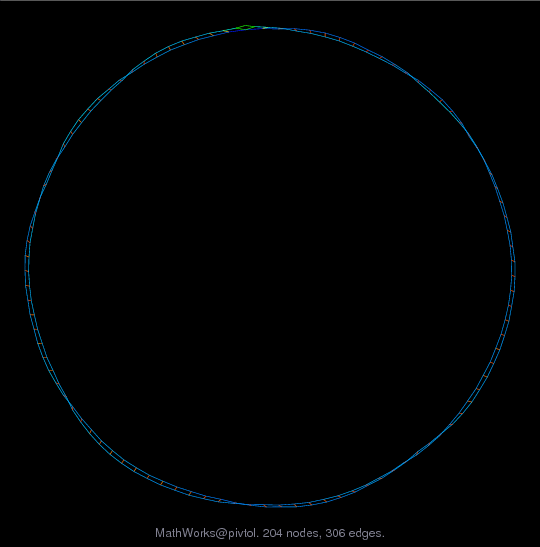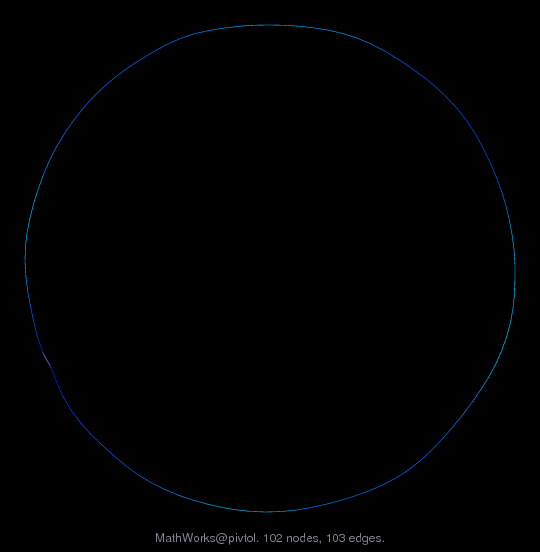Matrix: MathWorks/pivtol

Description: Spline toolbox. Pivot tol 0.1 fails, needs >= 0.26. MathWorks,Inc(bipartite graph drawing) (graph drawing of A+A')• Matrix group: MathWorks
• download as a MATLAB mat-file, file size: 3 KB. Use UFget(861) or UFget('MathWorks/pivtol') in MATLAB.

 Matrix properties number of rows 102 number of columns 102 nonzeros 306 structural full rank? yes structural rank 102 # of blocks from dmperm 1 # strongly connected comp. 1 explicit zero entries 0 nonzero pattern symmetry 99% numeric value symmetry 0% type real structure unsymmetric Cholesky candidate? no positive definite? no

 author B. Cheng editor T. Davis date 2002 kind statistical/mathematical problem 2D/3D problem? no

 Additional fields size and type b full 102-by-3

 Ordering statistics: result nnz(chol(P*(A+A'+s*I)*P')) with AMD 303 Cholesky flop count 9.0e+02 nnz(L+U), no partial pivoting, with AMD 504 nnz(V) for QR, upper bound nnz(L) for LU, with COLAMD 304 nnz(R) for QR, upper bound nnz(U) for LU, with COLAMD 500

 SVD-based statistics: norm(A) 1.41607 min(svd(A)) 0.0129195 cond(A) 109.607 rank(A) 102 sprank(A)-rank(A) 0 null space dimension 0 full numerical rank? yes

 singular values (MAT file): click here SVD method used: s = svd (full (A)) ; status: ok